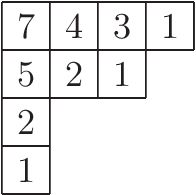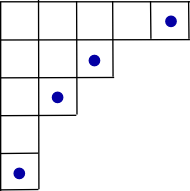UPD 2021.7.5: 感谢 Tiw_Air_OAO 大佬提醒，钩长公式有利用 LGV 引理的神奇证法，在 2019 年集训队论文里有提到。不过菜鸡博主还没看懂/kk，以后如果有机会弄明白的话会来更新一下。(1) 首先从 $n$ 个格子中随机选择一个作为起点，开始游走。
(2) 如果当前在角落格子，那么游走结束；否则，从该格正右方和正下方的 $\frac{1}{h_{i,j}-1}$ 个格子中等概率随机选择一个，跳到所选的点，继续该步骤。

$p_{a,b}=\frac{H(A-(a,b))}{H(A)}$

$f(a,b,c,d,S_1,S_2)=\frac{1}{h_{c,d}-1}\cdot (f(a,b,S_1,d,S_1[2..m_1],S_2)+f(a,b,c,S_2,S_1,S_2[2..m_2]))$

$f(a,b,S_1,d,S_1[2..m_1],S_2)=(h_{c,b}-1)\cdot P,f(a,b,c,S_2,S_1,S_2[2..m_2])=(h_{a,d}-1)\cdot P$

$f(a,b,c,d,S_1,S_2)=\frac{1}{h_{c,d}-1}(h_{c,b}-1+h_{a,d}-1)\cdot P$

$\frac{1}{n}\cdot \prod^{a-1}_{i=1}(1+\frac{1}{h_{i,b}-1})\cdot \prod^{b-1}_{j=1}(1+\frac{1}{h_{c,j}-1})$